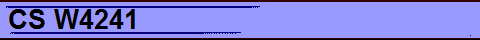CS W4241 - Numerical Algorithms and Complexity Spring 2013 Monday, Wednesday, 4:10 - 5:25 pm Professor: Joseph F. Traub Office Hours: TBA TA: TBA Grading: Midterm 30% Final 40% Homework 30% Extra credit homework 10% TOTAL 110% The course consists of two parts, complexity and algorithms. PART I - COMPLEXITY Rather than a text I´ll give you handouts. The following is an indication of the topics I´ll cover. Overview Integration Example Breaking the Curse Mathematical Finance Model of Computation Formal Models and Scientific Knowledge Complexity of Linear Programming Complexity of Verification Clock Synchronization in Distributed Networks Assigning Values to Mathematical Hypotheses OPTIONAL MATERIAL General Formulation of Information-Based Complexity Integration Example Concluded Value of Information in Computation A number of additional complexity topics will be covered in the lectures. There will be handouts for this material also. Topics include Playing 20 questions against a liar Continuous binary search Fast matrix multiplication Fast Fourier transform (FFT) Polynomial Evaluation Effect of precomputation PART II - ALGORITHMS The material on algorithms will be covered by handouts. Nonlinear Equations Univariate Multivariate Polynomial zeros Bernoulli algorithm Jenkins - Traub algorithm Randomization High dimensional integration  Numerical solution of partial differential equations Elliptic equations Parabolic equations Hyperbolic equations   Applications to science, engineering, finance.